Caribou
SofaCaribou::ode::BackwardEulerODESolver Class Reference

## Detailed Description

Implementation of an implicit backward euler solver compatible with non-linear materials.

We are trying to solve to following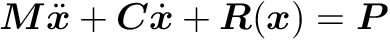Where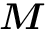is the mass matrix,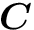is the damping matrix,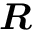is the (possibly non-linear) internal elastic force residual and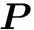is the external force vector (for example, gravitation force or surface traction).

We first transform this second-order differential equation to a first one by introducing two independant variables: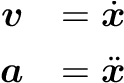Using the backward Euler scheme, we pose the following approximations: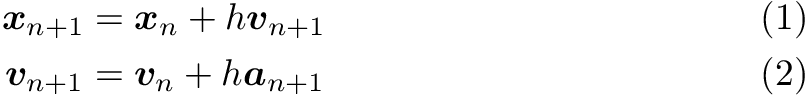where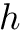is the delta time between the steps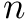and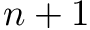.

Substituting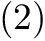inside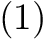gives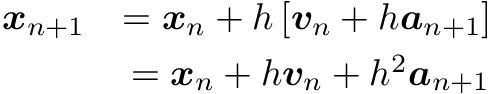And the problem becomes: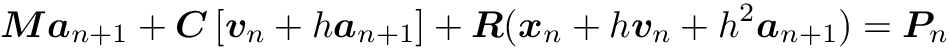where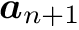is the vector of unknown accelerations.

Finally, assumingis non-linear in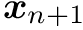, we iteratively solve forusing the Newton-Raphson method and using the previous approximations to back propagate it inside the velocity and position vectors.

Let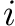be the Newton iteration number for a given time step, we pose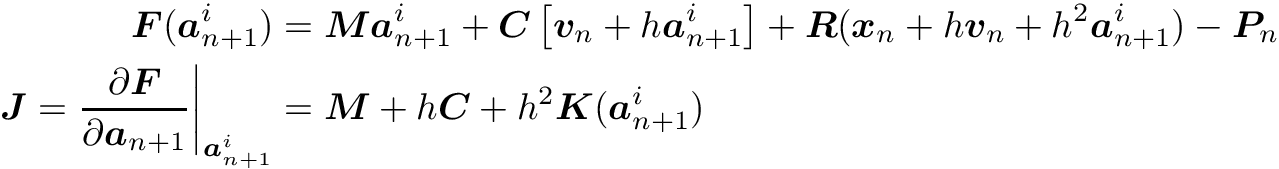where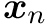andare the position and velocity vectors at the beginning of the time step, respectively, and remains constant throughout the Newton iterations.

We then solve for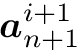with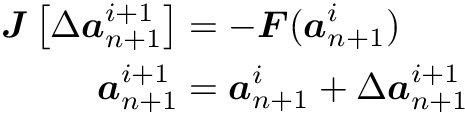And propagate back the new acceleration usingand.

In addition, this component implicitly adds a Rayleigh's damping matrix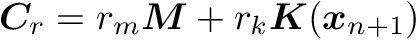. We therefore have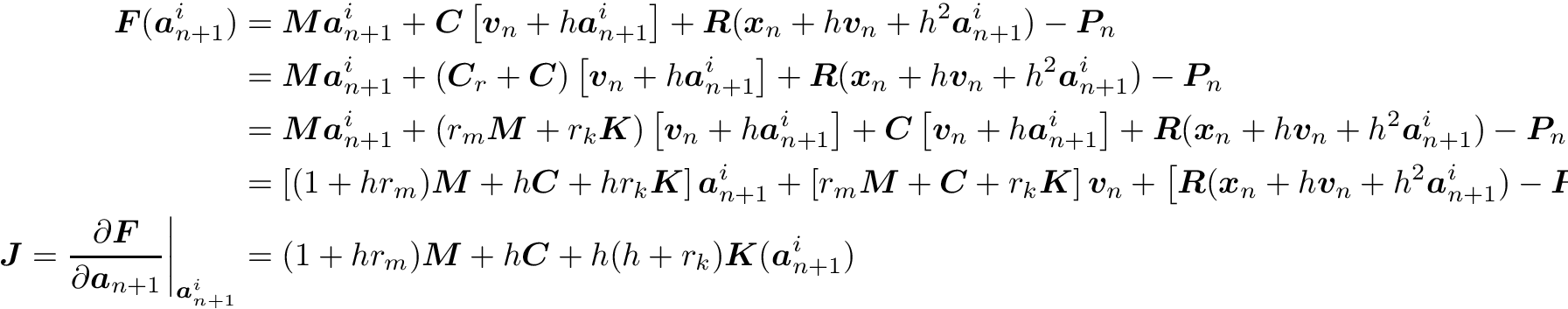#include <BackwardEulerODESolver.h>

Inheritance diagram for SofaCaribou::ode::BackwardEulerODESolver: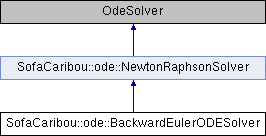## Public Types

template<typename T >
using Data = sofa::core::objectmodel::Data< T >Public Types inherited from SofaCaribou::ode::NewtonRaphsonSolver
template<typename T >
using Data = sofa::core::objectmodel::Data< T >

template<typename T >

## Public Member Functions

SOFA_CLASS (BackwardEulerODESolver, NewtonRaphsonSolver)

void solve (const sofa::core::ExecParams *params, SReal dt, sofa::core::MultiVecCoordId x_id, sofa::core::MultiVecDerivId v_id) overridePublic Member Functions inherited from SofaCaribou::ode::NewtonRaphsonSolver
SOFA_CLASS (NewtonRaphsonSolver, sofa::core::behavior::OdeSolver)

void init () override

void solve (const sofa::core::ExecParams *params, SReal dt, sofa::core::MultiVecCoordId x_id, sofa::core::MultiVecDerivId v_id) override

auto iteration_times () const -> const std::vector< UNSIGNED_INTEGER_TYPE > &
List of times (in nanoseconds) that each Newton-Raphson iteration took to compute in the last call to Solve().

auto squared_residuals () const -> const std::vector< FLOATING_POINT_TYPE > &
The list of squared residual norms (||r||^2) of every newton iterations of the last solve call.

auto squared_initial_residual () const -> const FLOATING_POINT_TYPE &
The initial squared residual (||r0||^2) of the last solve call.

The documentation for this class was generated from the following files:
• /home/jnbrunet/sources/caribou/src/Plugin/Ode/BackwardEulerODESolver.h
• /home/jnbrunet/sources/caribou/src/Plugin/Ode/BackwardEulerODESolver.cpp